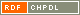# Show document

Title: Classification of 2-arc-transitive dihedrants Du, Shao Fei (Author)Malnič, Aleksander (Author)Marušič, Dragan (Author)http://dx.doi.org/10.1016/j.jctb.2008.02.007 English Not categorized 1.01 - Original Scientific Article IAM - Andrej Marušič Institute A complete classification of 2-arc-transitive dihedrants, that is, Cayley graphs of dihedral groups is given, thus completing the study of these graphs initiated by the third author in [D. Marušič, On 2-arc-transitivity of Cayley graphs, J. Combin. Theory Ser. B 87 (2003) 162-196]. The list consists of the following graphs: (i) cycles ▫$C_{2n},\; n \ge 3$▫; (ii) complete graphs ▫$K_{2n}, \; n \ge 3$▫; (iii) complete bipartite graphs ▫$K_{n,n}, \; n \ge 3$▫; (iv) complete bipartite graphs minus a matching ▫$K_{n,n} - nK_2, \; n \ge 3$▫; (v) incidence and nonincidence graphs ▫$B(H_{11})$▫ and ▫$B'(H_{11})$▫ of the Hadamard design on 11 points; (vi) incidence and nonincidence graphs ▫$B(PG(d,q))$▫ and ▫$B'(PG(d,q))$▫, with ▫$d \ge 2$▫ and ▫$q$▫ a prime power, of projective spaces; (vii) and an infinite family of regular ▫${\mathbb{Z}}_d$▫-covers ▫$K_{q+1}^{2d}$▫ of ▫$K_{q+1, q+1} - (q+1)K_2$▫, where ▫$q \ge 3$▫ is an odd prime power and ▫$d$▫ is a divisor of ▫$\frac{q-1}{2}$▫ and ▫$q-1$▫, respectively, depending on whether ▫$q \equiv 1 \pmod{4}$▫ or ▫$q \equiv 3 \pmod{4}$▫ obtained by identifying the vertex set of the base graph with two copies of the projective line ▫$PG(1,q)$▫, where the missing matching consists of all pairs of the form ▫$[i,i']$▫, ▫$i \in PG(1,q)$▫, and the edge ▫$[i,j']$▫ carries trivial voltage if ▫$i=\infty$▫ or ▫$j=\infty$▫, and carries voltage ▫$\bar{h} \in {\mathbb{Z}}_d$▫, the residue class of ▫$h \in {\mathbb{Z}}_d$▫, if and only if ▫$i-j = \theta^h$▫, where ▫$\theta$▫ generates the multiplicative group ▫${\mathbb{F}}_q^\ast$▫ of the Galois field ▫${\mathbb{F}}_q$▫. permutation group, imprimitive group, dihedral group, Cayley graph, dihedrant, 2-Arc-transitive graph 2008 str. 1349-1372 Vol. 98, iss. 6 0095-8956 519.17 20182771554 46Document is not linked to any category.

Average score: (0 votes) Voting is allowed only to logged in users.Hover the mouse pointer over a document title to show the abstract or click on the title to get all document metadata.